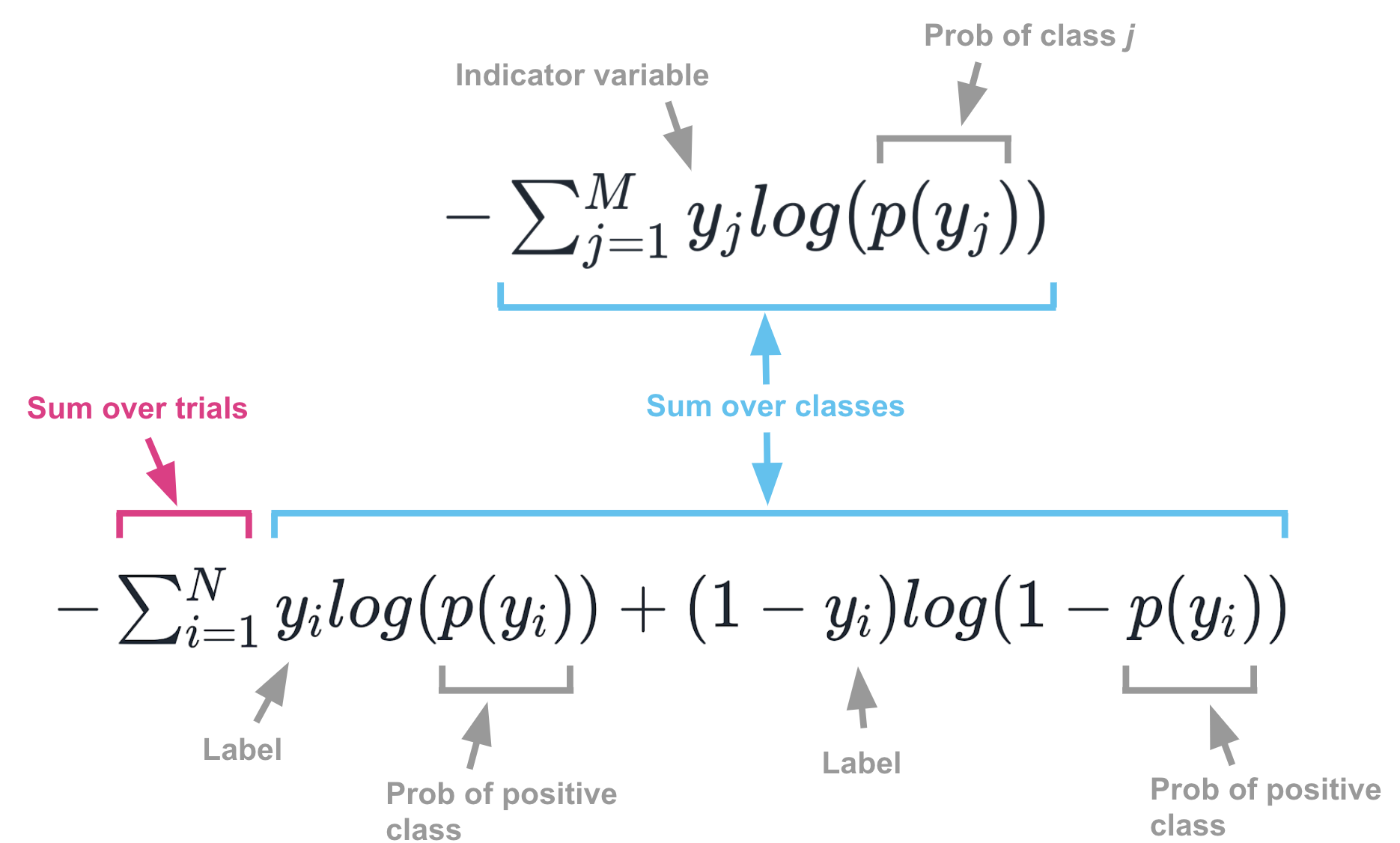# Two things that confused me about cross-entropy

Every once in a while, I try to better understand cross-entropy by skimming over some Medium posts and StackExchange answers. I always come away only half-understanding it.

A major cause of confusion is that different sources use different notations and conventions. Here are two that have tripped me up.

### p and q vs y and p

In information theory, the cross-entropy for an event with $$M$$ discrete outcome classes is

$H(p, q) = -\sum_{j=1}^M{p_j log (q_j)}$

… where $$p$$ is the true distribution of outcomes and $$q$$ is the approximating distribution.

But in machine learning, the cross-entropy is defined as:

$H(y, p) = -\sum_{j=1}^M{y_j log (p(y_j))}$

… where $$y$$ are the true labels and $$p(y)$$ is the approximating distribution, i.e. the classifier’s probability predictions.

Notice that the meaning of $$p$$ has been reversed! In information theory, it is the true distribution. In machine learning, it is the approximating distribution.

This points to a key difference between generic information theory and machine learning applications. In information theory, the target distribution $$p$$ is a probability distribution where the probability mass can be distributed broadly over the classes. In machine learning, the target distribution $$y$$ has all of its mass allocated to a known label.

### Multiple classes vs multiple trials

As shown above, the cross-entropy for a single event with $$M$$ classes is:

$H(y, p) = -\sum_{j=1}^M{y_j log (p(y_j))}$

If there are multiple trials, you just need to sum up the cross-entropies from all the trials so that the total cross-entropy is:

$H(y, p) = -\sum_{i=1}^N \sum_{j=1}^M{y_j^{(i)} log (p(y_j^{(i)}))}$

… where $$N$$ is the number of trials.

This all makes notational sense. Unfortunately, confusion arises when people use non-compact notation for summing over binary classes. When dealing with binary classification, it’s common to see cross-entropy defined as:

$H(y, p) = -\sum_{i=1}^N{y_i log (p(y_i)) + (1-y_i) log (1 -p(y_i))}$

Superficially, this looks a lot like the first formula, but it’s actually just a clever way of writing the second formula for binary classes. The first and third formulas confusingly look very similar, but the confusion goes away when you realize the two summation signs mean different things. One sums over classes, while the other sums over trials.

The image below shows these two formulas broken into their components.### Where to learn about cross-entropy

I don’t know if there is a single best place to learn about cross-entropy, but below are a few places that were helpful. If you read them make sure you think about (a) which notation they are using (b) whether they are writing about single trials or multiple trials and (c) whether they are writing about binary classes or multi classes.

• Statistical Rethinking. Information theory notation, single trial case, multiple classes.
• Andrew Webb. Both notations, both the single and multiple trial case, multiple classes.
• Harshith. ML notation, multiple trials, binary classes.